# Forces Acting on a Dam Structure and CalculationsReading time: 1 minute

Different types of forces acts on a dam structure such as water pressure, self weight, wave pressure etc.  Determination of various forces which acts on the structure is the first step in the design of dams. These forces are considered to act per unit length of the dam.

## Forces Acting on a Dam Structure

1. Water pressure
2. Uplift pressure or seepage loads
3. Earthquake forces
4. Self weight of the dam
5. Silt pressure
6. Wave pressure
7. Ice pressure

## 1. Water Pressure

It is the pressure of water that acts perpendicular on the upstream face of the dam. For this, there are two cases: A. Upstream face of the dam is vertical and there is no water on the downstream side of the dam Fig.1.Fig. 1:water pressure on the vertical upstream of a dam

The total pressure is in horizontal direction and acts on the upstream face at a height H/3 from the bottom. The water pressure on the dam is computed according to equation 1.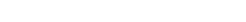Where: w: specific weight of water. Usually it is taken as unity. H: height up to which water is stored in m. B. Upstream face with batter and there is no water on the downstream side as shown in Fig.2.Fig.2: water pressure on the inclined upstream of a dam

Here in addition to the horizontal water pressure of equation 1, there is vertical pressure of the water. It is due to the water column resting on the upstream sloping side. The vertical pressure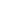acts on the length ‘b’ portion of the base. This vertical pressure is is calculated as follow: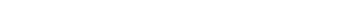Pressureacts through the center of gravity of the water column resting on the sloping upstream face. If there is water standing on the downstream side of the dam, water pressure will have vertical and horizontal component which can be using equation 1 except water height which is illustrated in Fig.3. The water pressure on the downstream face actually stabilizes the dam. Hence as an additional factor of safety, it may be neglected.Fig.3:water pressure at down stream of a dam structure

## 2. Uplift pressure or Seepage Loads

When the water is stored on the upstream side of a dam there exists a head of water equal to the height up to which the water is stored. This water enters the pores, fissures, and cracks of the foundation material under pressure. It also enters the joint between the dam and the foundation at the base and the pores of the dam itself. This water then seeps through and tries to emerge out on the downstream end. The seeping water creates hydraulic gradient between the upstream and downstream side of the dam. This hydraulic gradient causes vertical upward pressure. The upward pressure is known as uplift which is the second largest external pressure. Uplift reduces the effective weight of the structure and consequently the restoring force is reduced. Therefore, it is essential to study the nature of uplift and also some methods will have to be devised to reduce the uplift pressure value.Fig.4:uplift pressure

With reference to figure 3, uplift pressure is given by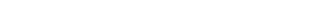Where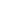is the uplift pressure, B is the base width of the dam and H is the height up to which water is stored. This total uplift acts at B/3 from the heel or upstream end of the dam. Uplift is generally reduced by constructing drainage pipes between dam and its foundation, constructing cut off walls under the upstream face, holes in the dam section, or pressure grouting the dame foundation.

## 3. Earthquake Forces

Dynamic loads created due to earthquakes must be considered in the design of all major dams located in ‘high-risk’ seismic regions, and for dams situated in close proximity to potentially active geological fault complexes. Earthquake produces waves in every possible direction. However, it has to be resolved into vertical and horizontal components for the design purposes. The horizontal component has greater effect. Seismic vibration influence both dam body and water in the reservoir of the dam. So, the generated dynamic loads are due to the inertia of the dam and hydrodynamic forces by the water in the reservoir.  Seismic forces are estimated according to the following equations:

### Forces due to inertia of the dam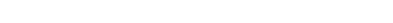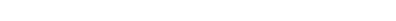where: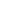and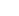: horizontal and vertical seismic coefficient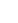: weight of the dam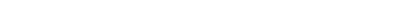where: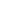: soil foundation factor, and taken as 1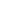: importance factor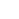: basic seismic coefficient taken from seismic maps.is taken as 1.5*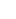andis taken as 0.75*. NOTE: Inertia forces are considered to operate through the centroid of the dam section.Fig.5:forces due to Inertia of the dam

### Hydrodynamic force due to inertia of water

Theoretical calculations indicate that the distribution of hydrodynamic pressure due to an earthquake on the upstream face of dam is nearly parabolic as shown in Fig.6.Fig.6: hydrodynamic force due to inertia of water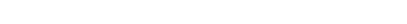Where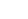:horizontal pressure H : depth of the reservoir c : computed using the following equation: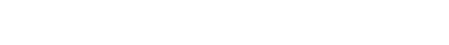where: z: depth of water in meters from the top of the reservoir to the point of consideration Cm: depends on the upstream slope Cm= 0.73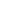/90: angle in degrees that the upstream slope makes with the horizontal. If the vertical portion is more than half the depth, the entire face is taken vertical; When the vertical portion is less than half the depth, the slope of the face is given by the line joining the heel to the water surface level at the upstream face as illustrated in Fig.7.Fig.7:upstream slope simplification

The total pressure force, Fe , on the portion of the dam up to depth z from the top and the moment, Me, about the heel of the plane up to which pressure is taken are respectively given by: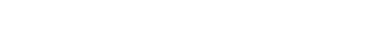## 4. Self-Weight of Dam

The weight of dam and its foundation is a major resisting force. It can be computed using the following equation: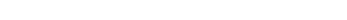Where: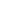: unit weight of dam material

## 5. Silt Pressure

The weight of dam and its foundation is a major resisting force. It act at h/3 from the base and can be computed using equation 12: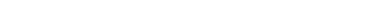Where: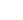: coefficient of active earth pressure of silt which equal to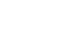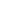: angle of internal friction of soil, cohesion neglected.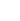: submerged unit weight of silt material. h: height of silt deposited.Fig.8:silt pressure on a dam

## 6. Wave Pressure

Waves are generated on the surface of the reservoir by the blowing winds, which exert a pressure on the upper part of the dam above the water level. This pressure is calculated using equation 13.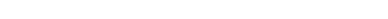Wave pressure depends upon wave height which is given by: For F < 32 km: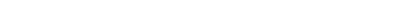For F > 32 km: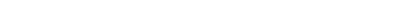Where: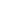: height of water from the top of crest to bottom of trough in meters. V: wind velocity in km/hour F: fetch or straight length of water expanse in km. The maximum pressure intensity due to wave action occurs when it acts at 0.5meters above the still water surface. Total force due to wave action is given by: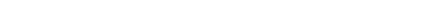Pw acting at 3/8above the reservoir surface.Fig.9:wave pressure on a dam

## 7. Ice Pressure

The ice which may be formed on the water surface of the reservoir in cold countries may sometimes melt and expand. The dam face is subjected to the thrust and exerted by the expanding ice. This force acts linearly along the length of the dam and at the reservoir level. The magnitude of this force varies from 250 to 1500 kN/sq.m depending upon the temperature variations. On an average, a value of 500 kN/sq.m may be taken under ordinary circumstances.